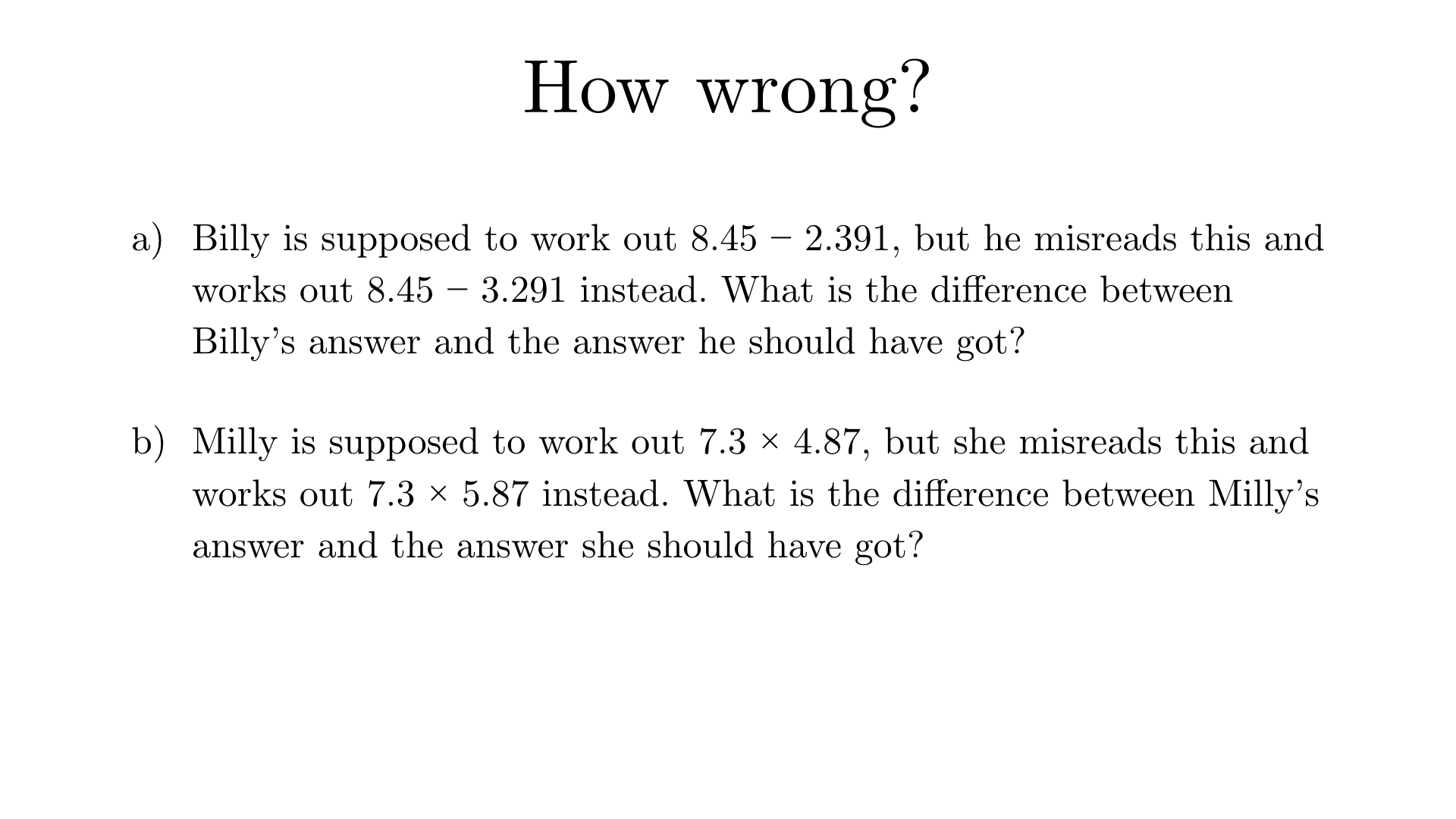Solution to (a)
There is no need to perform three separate subtractions. The difference will simply be the difference between 3.291 and 2.391 i.e. it will be 0.9.
Solution to (b)
There is no need to perform two separate multiplications followed by a subtraction. The difference will simply be 7.3, because 5.87 lots of 7.3 is 1 lot of 7.3 more than 4.87 lots of 7.3.